### Computing DeBroglie Wavelengths

We usually quote the energy of a particle in terms of its kinetic energy in electron Volts, eV (or Million electron Volts, MeV). The reason for this is that particles are usually accelerated to some energy by an electric field. If I let an electron (or proton...) be accelerated through a 100 Volt potential difference, it will have a kinetic energy of 100eV.

The whole problem of computing a deBroglie wavelength is to convert from kinetic energy to momentum. If you always want to be correct without any need for thinking, use the relativistically correct formula for the kinetic energyand solve it for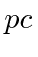,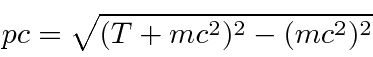then use this handy formula to get the answer.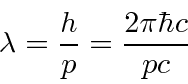I remember thatallowing me to keep the whole calculation in eV. I also know the masses of the particles.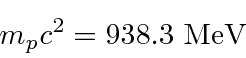(If, make sure the precision of your calculator sufficient or use the non-relativistic method below.)

If you know that the particle is super-relativistic, so that, then just useand life is easy.

If you know that the particle is highly non-relativistic,, then you can use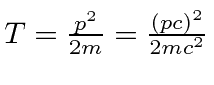giving.

So, for example, compute the wavelength of a 100 eV electron. This is non-relativistic since 100 eV « 510000 eV. So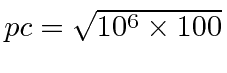eV or 10000 eV.Jim Branson 2013-04-22